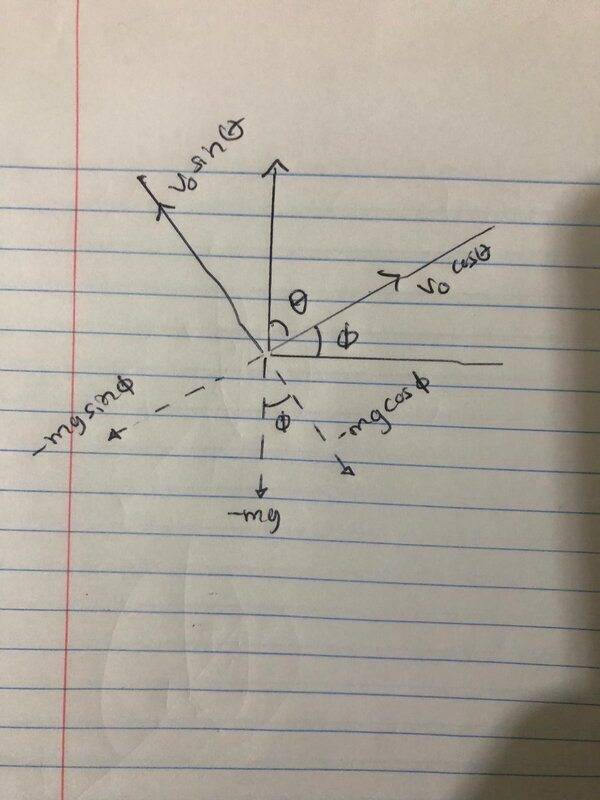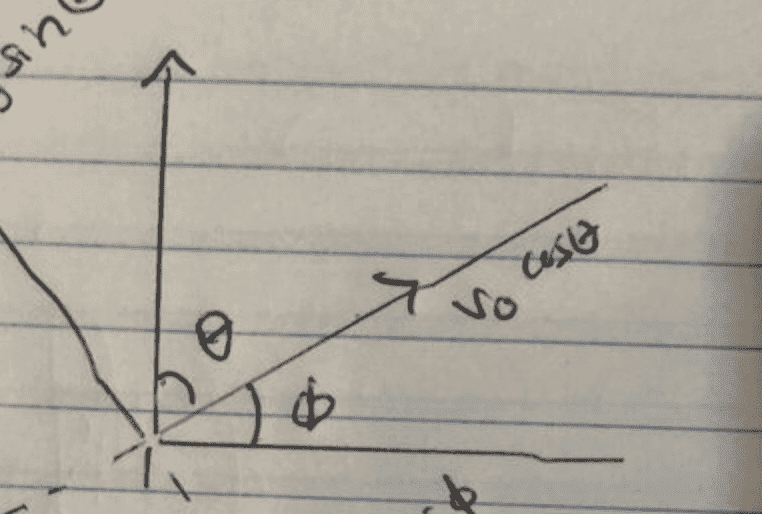# A ball thrown at an angle above an inclined plane

tryingtolearn1
Homework Statement:
Suppose a ball is thrown straight up with an initial speed of ##v_i## on an inclined plane, where the plane is inclined at an angle of ##\phi## above the horizontal and the ball's initial velocity is at an angle ##\theta## above the plane.
Relevant Equations:
##F=ma##
I understand how the diagram below determined the ##x## and ##y## axis for the velocity vectors but I don't understand the gravity vectors. What I don't understand about the gravity vectors is why is ##-mg## in the ##y-##axis equal to ##-mg\cos\theta## and the ##x-##axis is equal to ##-mg\cos\theta## even though the diagram for the velocity vector has the ##y-##axis as ##v_0\sin\theta## which is exactly on the same axis for the gravity vector so shouldn't the gravity vectors ##\cos## and ##\sin## be flipped? Also, I don't understand how the ##\phi## angle below the horizontal was determined in the diagram. Shouldn't that ##\phi## be instead ##\theta##?Homework Helper
Gold Member
Homework Statement:: Suppose a ball is thrown straight up with an initial speed of ##v_i## on an inclined plane, where the plane is inclined at an angle of ##\phi## above the horizontal and the ball's initial velocity is at an angle ##\theta## above the plane.
Relevant Equations:: ##F=ma##

I understand how the diagram below determined the ##x## and ##y## axis for the velocity vectors but I don't understand the gravity vectors. What I don't understand about the gravity vectors is why is ##-mg## in the ##y-##axis equal to ##-mg\cos\theta## and the ##x-##axis is equal to ##-mg\cos\theta## even though the diagram for the velocity vector has the ##y-##axis as ##v_0\sin\theta## which is exactly on the same axis for the gravity vector so shouldn't the gravity vectors ##\cos## and ##\sin## be flipped? Also, I don't understand how the ##\phi## angle below the horizontal was determined in the diagram. Shouldn't that ##\phi## be instead ##\theta##?

View attachment 269459
There is no ##mg\cos\theta## in the diagram. The weight ##mg## down is correctly resolved into components where the ##x##-axis is up the incline and the ##y##-axis perpendicular and away from the incline and ##\phi## is the angle of the incline. Note that ##\theta## is complementary to ##\phi## so that ##\cos\phi= \sin\theta## and ##\sin\phi=\cos\theta.##

Angle ##\phi## below the horizontal is equal to angle ##phi## above the horizontal because they have their sides mutually perpendicular. That's a theorem from geometry. You can see why that is if you imagine the angle ##phi## above the horizontal being decreased to zero in which case angle ##\phi## below the horizontal will correspondingly go to zero.

Last edited:
tryingtolearn1
There is no ##mg\cos\theta## in the diagram. The weight ##mg## down is correctly resolved into components where the ##x##-axis is up the incline and the ##y##-axis perpendicular and away from the incline and ##\phi## is the angle of the incline. Note that ##\theta## is complimentary to ##\phi## so that ##\cos\phi= \sin\theta## and ##\sin\phi=\cos\theta.##

Angle ##\phi## below the horizontal is equal to angle ##phi## above the horizontal because they have their sides mutually perpendicular. That's a theorem from geometry. You can see why that is if you imagine the angle ##phi## above the horizontal being decreased to zero in which case angle ##\phi## below the horizontal will correspondingly go to zero.

Ops, I meant to say ##mg\cos\phi## and ##mg\sin\phi##, not ##mg\cos\theta## and ##mg\sin\theta## . Why are they complimentary even though that in the diagram is clear that ##v_0\sin\theta## and ##-mg\cos\phi## are on the same axis so shouldn't they both have the same trig function of ##\sin##?

Also, do you know of the name of the geometrical theorem?

Homework Helper
Gold Member
Ops, I meant to say ##mg\cos\phi## and ##mg\sin\phi##, not ##mg\cos\theta## and ##mg\sin\theta## . Why are they complimentary even though that in the diagram is clear that ##v_0\sin\theta## and ##-mg\cos\phi## are on the same axis so shouldn't they both have the same trig function of ##\sin##?

Also, do you know of the name of the geometrical theorem?
Complementary means ##\theta +\phi=90^o.## It sure looks like it in your diagram.As I said before, ##v_0\sin\theta=v_0\cos\phi## because the angles are complimentary, so they do have the same trig function.

I do not think that the theorem has a name. It was proven by Euclid who lived 23 centuries ago. It suffices to say that "two acute angle that have their sides mutually perpendicular are equal." It's a good one to remember.

Last edited:
•tryingtolearn1 and Lnewqban
Homework Helper
Gold Member
What is the reason for the inclined plane to be?
The trajectory of the ball is going to be perfectly vertical, regardless the angles shown.
Is this part of a problem that has more questions or conditions?

Last edited:
Homework Helper
Gold Member
What is the reason for the inclined plane to be?
The trajectory of the bal is going to be perfectly vertical, regardles the angles shown.
Is this part of a problem that has more questions or conditions?
Only part of the problem was provided, not the whole thing or the question to be addressed, therefore I cannot speculate about the inclined plane's raison d'être.

Last edited:
tryingtolearn1
Ty @kuruman .

@Lnewqban it is just part of the problem and not the whole question. I just needed clarificaiton of the diagram which is why I didn't write the whole question.

Homework Helper
Gold Member
Ty @kuruman .

@Lnewqban it is just part of the problem and not the whole question. I just needed clarificaiton of the diagram which is why I didn't write the whole question.
Thank you.Homework Helper
Gold Member
2022 Award
Homework Statement:: Suppose a ball is thrown straight up with an initial speed of ##v_i## on an inclined plane, where the plane is inclined at an angle of ##\phi## above the horizontal and the ball's initial velocity is at an angle ##\theta## above the plane.
Is this a translation? I suspect it is thrown 'straight up' in the sense that its trajectory is in a verical plane orthogonal to the plane of the slope. It is thrown at an angle θ+φ to the horizontal, not vertically.

•Lnewqban
tryingtolearn1
Is this a translation? I suspect it is thrown 'straight up' in the sense that its trajectory is in a verical plane orthogonal to the plane of the slope. It is thrown at an angle θ+φ to the horizontal, not vertically.
Yes, correct. I am unable to edit the question so I am unable to add to it but I should add that ##\theta > \phi##.

•